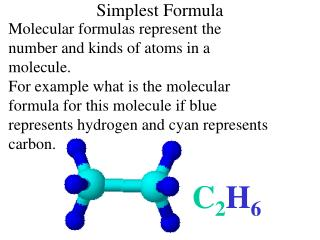DownloadDownload PresentationSimplest Formula

# Simplest Formula

Download Presentation## Simplest Formula

- - - - - - - - - - - - - - - - - - - - - - - - - - - E N D - - - - - - - - - - - - - - - - - - - - - - - - - - -
##### Presentation Transcript

1. Simplest Formula Molecular formulas represent the number and kinds of atoms in a molecule. For example what is the molecular formula for this molecule if blue represents hydrogen and cyan represents carbon. C2H6

2. Simplest Formula What is the molecular formula for this molecule if blue represents hydrogen and cyan represents carbon. C4H10

3. Simplest Formula It is useful for chemists to know the basic ratio of elements in a molecular formula. For instance for this molecule below the ratio of hydrogen to carbon is 6:2 or 3:1 C2H6

4. Simplest Formula What is the ratio of hydrogen to carbon in this molecule? 10 : 4 or simplified is 2.5 : 1 C4H10

5. What is the Simplest Formula? carbon hydrogen oxygen C6H12O6 CH2O

6. carbon chlorine hydrogen Simplest Formula is C2Cl4H2 CCl2H

7. carbon fluorine Simplest Formula is C4F10 C2F5 CF2.5 Simplest Formulas are always given whole number values

8. nitrogen oxygen simplest formula is NO2

9. Simplest formulas can be determined if the ratio, in moles, of each different element is known. What is the simplest formula of a substance which has a ratio of 2 mol of H, to 1 mol of S to 4 mol of O H2SO4

10. What is the simplest formula of a substance which has a ratio of 3.6 mol of H, to 1.2 mol of P to 4.8 mol of O Compare all elements to the element present in the smallest quantity. In this case P. Ratio of H to P is 3.6 mol H / 1.2 mol P = 3:1 Ratio of O to P is 4.8 mol O/ 1.2 mol P = 4:1 Simplest Formula is H3PO4

11. What is the simplest formula of a substance which has a ratio of 3.6 mol of H, to 3.6 mol of N to 7.2 mol of O Compare all elements to the element present in the smallest quantity. In this case N. Ratio of H to N is 3.6 mol H / 3.6 mol N = 1:1 Ratio of O to N is 7.2 mol O/ 3.6 mol N = 2:1 Simplest Formula is HNO2

12. What is the simplest formula of a substance which has a ratio of9.6 mol of Li, to 9.6 mol of Cr to 33.6 mol of O Compare all elements to the element present in the smallest quantity. In this case Li orCr. Ratio of O to Li is 33.6 mol O/ 9.6 molN=3.5:1 Simplest Formula is LiCrO3.5 Remember formulas are written with whole numbers, so double everything Li2Cr2O7

13. Percentage composition data can be used to determine the ratio, in moles, of each of the different elements present.

14. A substance is found to contain 50% S, and 50% O by mass. What is its simplest formula? In 100 g of this substance there are 50 g of S and 50 g of O. Find the number of moles, n, of S and O present in 50 g respectively. Ratio of O:S 3.13 mol O 50 g = 1.56 mol S nS = 32 g/mol 1.56 mol S = 2:1, SF is SO2 50 g = 3.13 mol O no = 16 g/mol

15. A substance is found to contain 56.4% O, and 43.6% P by mass. What is its simplest formula? In 100 g of this substance there are 56.4 g of Oand 43.6 g of P. Find the number of moles, n, of O and P present. Ratio of O:P 3.5 mol O 56.4 g = 3.5 mol O nO = 1.41 mol P = 2.5:1, SF is PO2.5 P2O5 16 g/mol 43.6 g = 1.41 mol P nP = 31 g/mol